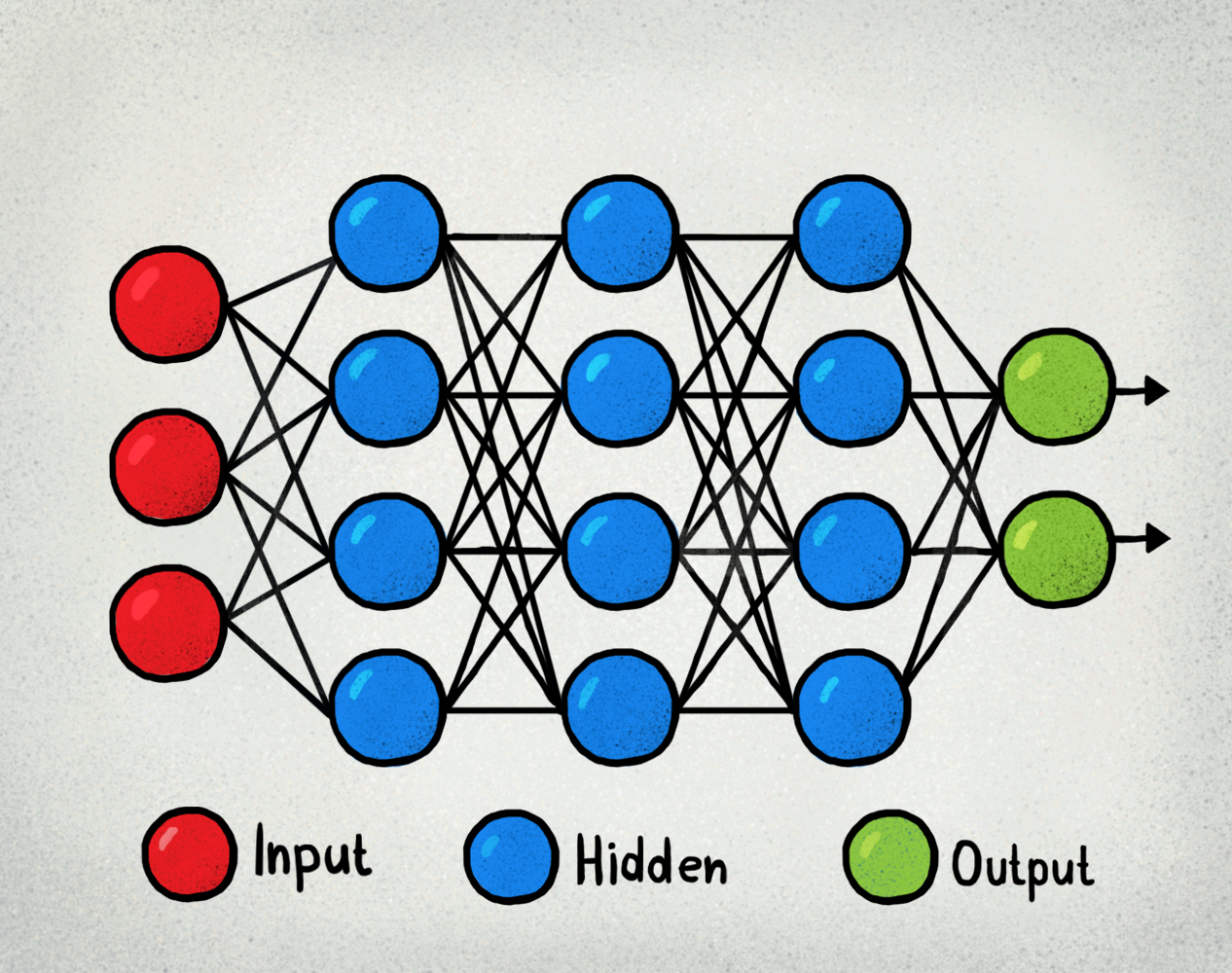# Types of Machine Learning Systems:

There are so many different types of Machine Learning systems that is:

Let’s discuss each of them in detail:

In supervised learning, the training data you feed to the algorithm includes the desired solutions, called labels.

A typical supervised learning task is classification. The spam filter is a good example of this: it is trained with many example emails along with their class (spam or ham), and it must learn how to classify new emails.

Another typical task is to predict a…

# Regularization in Deep Learning

There are 3 methods to avoid overfitting in Deep Learning:

## What is Regularization?

Regularization is a way to prevent overfitting.

There are two most common types of regularization used in training deep learning models. Which is L1 and L2.

Regularization: L1 & L2:

L1 regularizer: Cost function = Loss + λ…

# Implementation of LeNet From Scratch using Keras.

Here in this blog, I’m going to discuss the code implementation of LeNet using Keras. In this, I used this research for http://yann.lecun.com/exdb/publis/pdf/lecun-98.pdf for implementation.

LeNet-5 Total seven-layer does not comprise an input, each containing trainable parameters; each layer has a plurality of the Map the Feature, a characteristic of each of the input FeatureMap extracted by means of a convolution filter, and then each FeatureMap There is multiple neurons.

# CNN (Convolutional Neural Network)

Why CNN?

Convolution layers reduce the number of parameters and speed up the training of the model significantly.

CNN is basically a partially connected layer.

There are 3 types of layers in Convolutional Neural Network:

Here I’m going to discuss all of 3 layers in detail:

The first layer on CNN is the convolutional layer. It applies a filter to input to create a feature map that summarizes the presence of detected features in the input.

In the convolutional layer, the image became the stack of the filtered images and the number…

# Limitation of Feed-Forward Neural Network and CNN :

Issues with Feed-Forward Neural Network:

Issues with Convolutional Neural Network:

# Optimizers in Machine Learning

Optimizers are algorithms or methods used to change the attributes of the neural network such as weights and learning rates in order to reduce the losses.

Different types of optimizers:

Here I’m going to discuss all of them in detail:

BGD uses the data of the entire training set to calculate the gradient of the cost function to the parameters.

Because this method calculates the gradient for the entire data set in one update, the calculation…

# Weight Initialization in Neural NetworkThe key point that is taken care of before weight initialization:

Before Understanding the weight initialization firstly we have to understand fan_in and fan_out concept.

# Activation Function

Activation Function in Deep Learning helps to determine the output of the neural network. Also helps to normalize the output of each neuron.

Neural networks use non-linear activation functions, which can help the network learn complex data, compute and learn almost any function representing a question, and provide accurate predictions.

Here I’m going to discuss the different types of activation functions.

Now I’m going to discuss all of them in detail.

The sigmoid function is the most frequently used activation function at the beginning of Deep Learning.

In the…